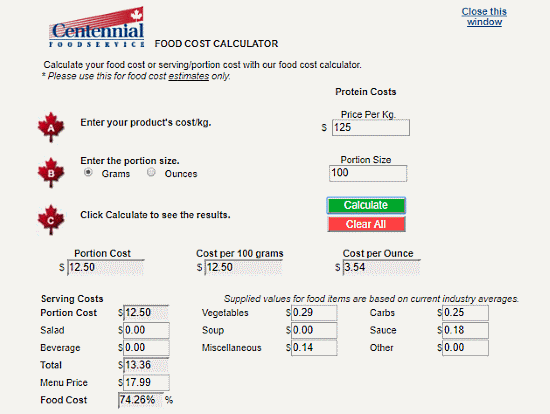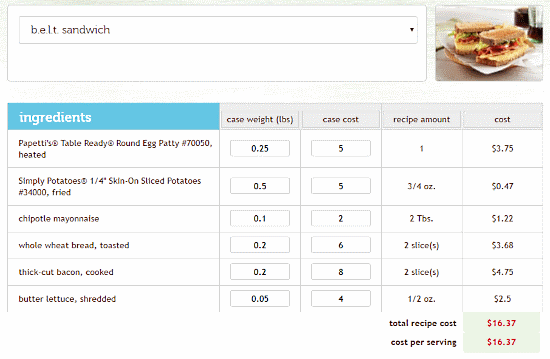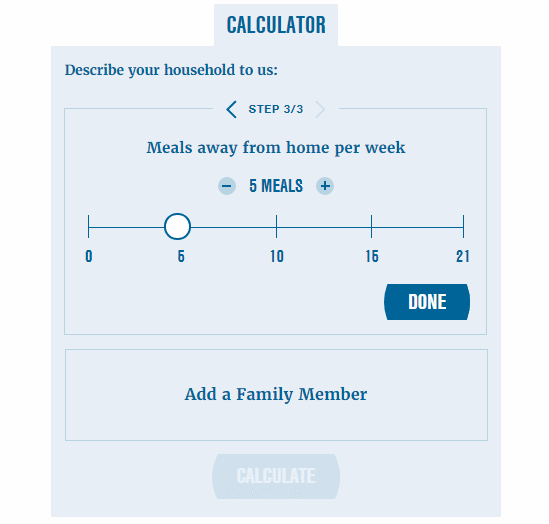Editor Ratings:
User Ratings:
[Total: 1 Average: 5]

With a food cost calculator, you can get a rough idea of how much you are spending on food. There are some food cost calculators that can calculate the actual cost of restaurant food items based on its ingredients cost.

Each of these websites offers a different type of food calculator. The first one lets you calculate the food cost based on each item’s price. Second and third help you calculate the cost per piece and serving per portion respectively. The fourth one helps you calculate a restaurant food cost by their ingredients. And, you can use the fifth one to estimate your weekly and monthly food cost.

Also read: 10 Free Online Calorie Calculator For Weight Loss

## Free Online Calculator UseThis Food Cost Calculator calculates the food cost based on individual item’s price. It helps you calculate the food cost and serving size cost percentage. You can break your whole order into portions then, you can calculate individual portion cost. You can easily calculate the cost of recipe or menu with this calculator. Simply enter the food items, cost, number of portions, cost per portions, etc. in the calculator. Based on your data, it will calculate the total food cost and food cost percentage for you. It also generates a food cost percentage report which you can print from here.

Cal this food cost calculator here.

## American Food GroupAmericanFoodGroup offers a cost per piece calculator to help you calculate the food cost and gross profit. Here, you enter the cost of a food item and units per case. Then, you can specify the per unit price and your serving size along with garnish cost and menu price. Based on all that data, this calculator calculates the cost per serving, total cost per plate and the profit you make per case. This calculator is designed for restaurants but individuals can also use it to break down their restaurant bills.

Calculate cost per piece with this food cost calculator here.

## Centennial Food ServiceThis Food Cost Calculator is similar to the previous one. It helps you calculate the food and serving costs. All you have to do is enter per Kg price of your main product and define its portion size (the size you serve per plate). Then, click the “Calculate” button to calculate the portion cost and food cost percentage. If you also serve other items like Salad, Beverage, etc., you can add them here. You can also specify the cost of ingredients and then calculate the total cost and cost percentage based on that.

Try this food cost calculator here.

## Explore Beyond BreakfastExploreBeyondBreakfast offers a food cost calculator that helps you determine food cost of recipes. With this calculator, you can calculate the ingredients cost, total recipe cost and cost per serving. To calculate a recipe cost here, simply select a food item from the menu which has over 60 different food items. Once you select a food item, this calculator lists all its ingredients. You can enter the case weight and case cost for each ingredient and calculate the ingredients cost for the selected food item. This calculator adds all ingredients’ costs and calculates the total recipe cost for you.

You can use this food cost calculator here.

## Spend SmartSpendSmart‘s food cost calculator helps you calculate your weekly and monthly food expenses. This is more like a food budget calculator for individuals and families. This calculator asks you how many meals you take away from home per week and calculate your weekly food expense based on the latest prices of USDA’s Low-Cost Food Plan. If you are living with your family, you can add your family member(s) and calculate your combined food expenses.

Calculate your food expenses with this food cost calculator here.

### Closing Words:

All these online food cost calculator websites are easy to use. You can use any of these to get an estimate of your food cost. You can calculate your weekly food expenses and plan accordingly. With ExploreBeyondBreakfast, you calculate food cost by ingredients and compare the price with the restaurant price.

 Editor Ratings: User Ratings:[Total: 1 Average: 5] Free/Paid: Free Tags: calculator